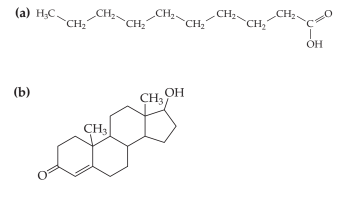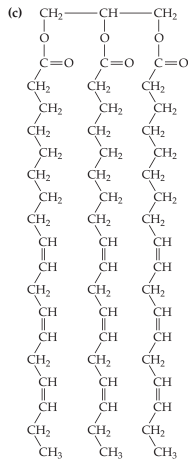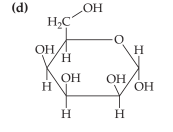×
Get Full Access to Introductory Chemistry - 5 Edition - Chapter 19 - Problem 53p
Get Full Access to Introductory Chemistry - 5 Edition - Chapter 19 - Problem 53p

×

Determine whether each molecule is a lipid. If the molecule is a lipid, determine theISBN: 9780321910295 34

Solution for problem 53P Chapter 19

Introductory Chemistry | 5th Edition

• Textbook Solutions
• 2901 Step-by-step solutions solved by professors and subject experts
• Get 24/7 help from StudySoup virtual teaching assistantsIntroductory Chemistry | 5th Edition

4 5 1 298 Reviews
27
1
Problem 53P

Determine whether each molecule is a lipid. If the molecule is a lipid, determine the kind of lipid. If it is a fatty acid or a triglyceride, classify it as saturated or unsaturated.Step-by-Step Solution:
Step 1 of 3

Solution:It is a lipid and the class is a saturated fatty acid

Step-2It is a lipid and the class is -steroid

Step-3It is an unsaturated  triglyceride.

Step-4It is a monosaccharides

Step 2 of 3

Step 3 of 3

ISBN: 9780321910295

This full solution covers the following key subjects: lipid, molecule, determine, acid, kind. This expansive textbook survival guide covers 19 chapters, and 2046 solutions. This textbook survival guide was created for the textbook: Introductory Chemistry, edition: 5. Since the solution to 53P from 19 chapter was answered, more than 781 students have viewed the full step-by-step answer. Introductory Chemistry was written by and is associated to the ISBN: 9780321910295. The answer to “?Determine whether each molecule is a lipid. If the molecule is a lipid, determine the kind of lipid. If it is a fatty acid or a triglyceride, classify it as saturated or unsaturated.” is broken down into a number of easy to follow steps, and 33 words. The full step-by-step solution to problem: 53P from chapter: 19 was answered by , our top Chemistry solution expert on 05/06/17, 06:45PM.

Unlock Textbook Solution